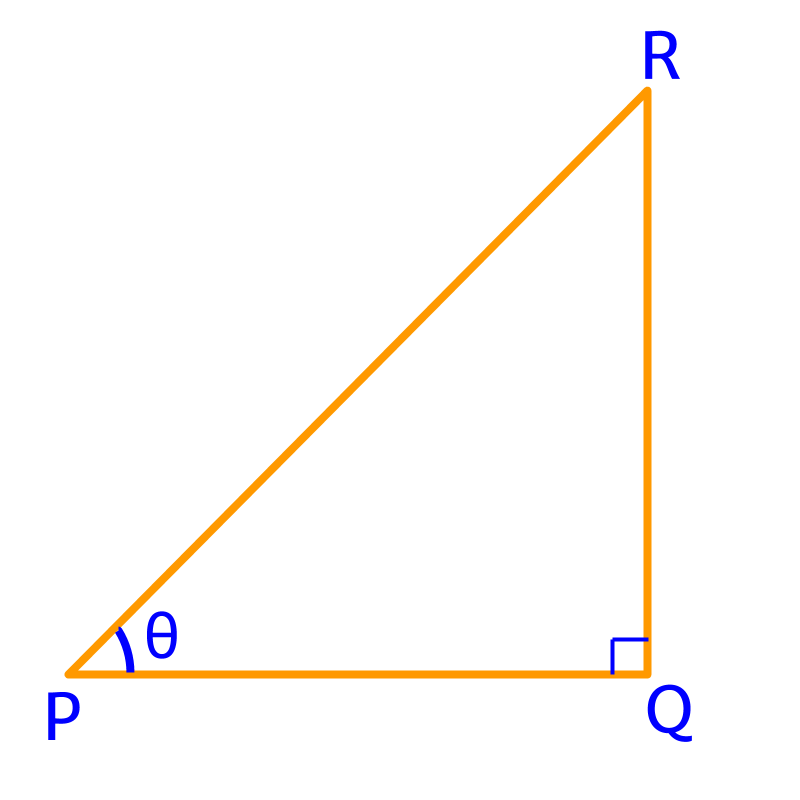maths > trigonometry

Trigonometric Ratios for Complementary Angles

what you'll learn...

Overview

»  Quickly follow that the adjacent and opposite sides swap for complementary angles... no need to memorize.

$\mathrm{sin}\left(90-\theta \right)=\mathrm{cos}\theta$$\sin \left(90 - \theta\right) = \cos \theta$
$\mathrm{cos}\left(90-\theta \right)=\mathrm{sin}\theta$$\cos \left(90 - \theta\right) = \sin \theta$
$\mathrm{tan}\left(90-\theta \right)=\mathrm{cot}\theta$$\tan \left(90 - \theta\right) = \cot \theta$

$\mathrm{csc}\left(90-\theta \right)=\mathrm{sec}\theta$$\csc \left(90 - \theta\right) = \sec \theta$
$\mathrm{sec}\left(90-\theta \right)=\mathrm{csc}\theta$$\sec \left(90 - \theta\right) = \csc \theta$
$\mathrm{cot}\left(90-\theta \right)=\mathrm{tan}\theta$$\cot \left(90 - \theta\right) = \tan \theta$

Proof

The complementary angle of $\theta$$\theta$ is $90-\theta$$90 - \theta$ or the angle that completes the given angle to a right angle.In the right angled triangle shown in figure, if $\angle P=\theta$$\angle P = \theta$ then

$\angle R=90-\theta$$\angle R = 90 - \theta$ which is the complementary angle of $\theta$$\theta$

In the right triangle shown in figure, the opposite side of $\angle P$$\angle P$ is $QR$$Q R$.

In the right triangle shown in figure, the adjacent side of $\angle R$$\angle R$ is $QR$$Q R$.

A right angled triangle is shown in figure.

Opposite of $\angle \theta$$\angle \theta$ = Adjacent of $\angle \left(90-\theta \right)$$\angle \left(90 - \theta\right)$
Adjacent of $\angle \theta$$\angle \theta$ = Opposite of $\angle \left(90-\theta \right)$$\angle \left(90 - \theta\right)$

The trigonometric ratios of $90-\theta$$90 - \theta$ can be expressed in terms of trigonometric ratios of $\theta$$\theta$ or vice versa.

From the figure
$\mathrm{sin}\theta =\frac{RQ}{PR}$$\sin \theta = \frac{R Q}{P R}$
$\mathrm{cos}\theta =\frac{PQ}{PR}$$\cos \theta = \frac{P Q}{P R}$
$\mathrm{sin}\left(90-\theta \right)=\frac{PQ}{PR}$$\sin \left(90 - \theta\right) = \frac{P Q}{P R}$
$\mathrm{cos}\left(90-\theta \right)=\frac{RQ}{PR}$$\cos \left(90 - \theta\right) = \frac{R Q}{P R}$

Comparing the above, it is concluded that
$\mathrm{sin}\left(90-\theta \right)=\mathrm{cos}\theta$$\sin \left(90 - \theta\right) = \cos \theta$
$\mathrm{cos}\left(90-\theta \right)=\mathrm{sin}\theta$$\cos \left(90 - \theta\right) = \sin \theta$

tan and cot

It is noted that the two angles in a right angles triangle are complementary angles and the trigonometric ratios of one angle are related to the trigonometric ratios of the other angle.

That is

•  hypotenuse is common for both the angles

•  opposite side for an angle is the adjacent side for the other angle

•  adjacent side for an angle is the opposite side for the other angle

This relation is used to derived Trigonometric Ratios for Complementary Angles .

Trigonometric Ratios for Complementary Angles:
$\mathrm{sin}\left(90-\theta \right)=\mathrm{cos}\theta$$\sin \left(90 - \theta\right) = \cos \theta$
$\mathrm{cos}\left(90-\theta \right)=\mathrm{sin}\theta$$\cos \left(90 - \theta\right) = \sin \theta$

The trigonometric ratios of $90-\theta$$90 - \theta$ can be expressed in terms of trigonometric ratios of $\theta$$\theta$ or vice versa.

From the figure
$\mathrm{tan}\theta =\frac{RQ}{PQ}$$\tan \theta = \frac{R Q}{P Q}$
$\mathrm{cot}\theta =\frac{PQ}{RQ}$$\cot \theta = \frac{P Q}{R Q}$
$\mathrm{tan}\left(90-\theta \right)=\frac{PQ}{RQ}$$\tan \left(90 - \theta\right) = \frac{P Q}{R Q}$
$\mathrm{cot}\left(90-\theta \right)=\frac{RQ}{PQ}$$\cot \left(90 - \theta\right) = \frac{R Q}{P Q}$

Comparing the above, it is concluded that
$\mathrm{tan}\left(90-\theta \right)=\mathrm{cot}\theta$$\tan \left(90 - \theta\right) = \cot \theta$
$\mathrm{cot}\left(90-\theta \right)=\mathrm{tan}\theta$$\cot \left(90 - \theta\right) = \tan \theta$

secant and cosecant

The trigonometric ratios of $90-\theta$$90 - \theta$ can be expressed in terms of trigonometric ratios of $\theta$$\theta$ or vice versa.

From the figure
$\mathrm{csc}\theta =\frac{PR}{RQ}$$\csc \theta = \frac{P R}{R Q}$
$\mathrm{sec}\theta =\frac{PR}{PQ}$$\sec \theta = \frac{P R}{P Q}$
$\mathrm{csc}\left(90-\theta \right)=\frac{PR}{PQ}$$\csc \left(90 - \theta\right) = \frac{P R}{P Q}$
$\mathrm{sec}\left(90-\theta \right)=\frac{PR}{RQ}$$\sec \left(90 - \theta\right) = \frac{P R}{R Q}$

Comparing the above, it is concluded that
$\mathrm{csc}\left(90-\theta \right)=\mathrm{sec}\theta$$\csc \left(90 - \theta\right) = \sec \theta$
$\mathrm{sec}\left(90-\theta \right)=\mathrm{csc}\theta$$\sec \left(90 - \theta\right) = \csc \theta$

examples

Does $\mathrm{sin}36$$\sin 36$ equal $\mathrm{sin}54$$\sin 54$ or $\mathrm{cos}54$$\cos 54$?
The answer is '$\mathrm{cos}54$$\cos 54$', as $\mathrm{sin}36$$\sin 36$$=\mathrm{sin}\left(90-54\right)$$= \sin \left(90 - 54\right)$ $=\mathrm{cos}54$$= \cos 54$

$\frac{\mathrm{tan}75}{\mathrm{cot}15}=?$
The answer is '$1$$1$' As $\mathrm{cot}15$$\cot 15$ $=\mathrm{tan}\left(90-15\right)$$= \tan \left(90 - 15\right)$ $=\mathrm{tan}75$$= \tan 75$.

Simplify $\mathrm{sin}36-\mathrm{cos}36+\mathrm{sin}54$$\sin 36 - \cos 36 + \sin 54$.
The answer is '$\mathrm{sin}36$$\sin 36$'.

Summary

Quickly follow that the adjacent and opposite sides swap for complementary angles... no need to memorize.

$\mathrm{sin}\left(90-\theta \right)=\mathrm{cos}\theta$$\sin \left(90 - \theta\right) = \cos \theta$

$\mathrm{cos}\left(90-\theta \right)=\mathrm{sin}\theta$$\cos \left(90 - \theta\right) = \sin \theta$

$\mathrm{tan}\left(90-\theta \right)=\mathrm{cot}\theta$$\tan \left(90 - \theta\right) = \cot \theta$

$\mathrm{csc}\left(90-\theta \right)=\mathrm{sec}\theta$$\csc \left(90 - \theta\right) = \sec \theta$

$\mathrm{sec}\left(90-\theta \right)=\mathrm{csc}\theta$$\sec \left(90 - \theta\right) = \csc \theta$

$\mathrm{cot}\left(90-\theta \right)=\mathrm{tan}\theta$$\cot \left(90 - \theta\right) = \tan \theta$

Outline

The outline of material to learn "Basics of Trigonometry" is as follows.

•   Detailed outline of "trigonometry".

→   Basics - Angles

→   Basics - Triangles

→   Importance of Right Angled Triangle

→   Trigonometric Ratio (Basics)

→   Triangular Form of Trigonometric Ratios

→   Introduction to Standard Angles

→   Trigonometric Ratio of Standard Angles

→   Trigonometric Identities

→   Trigonometric Ratios of Complementary Angles